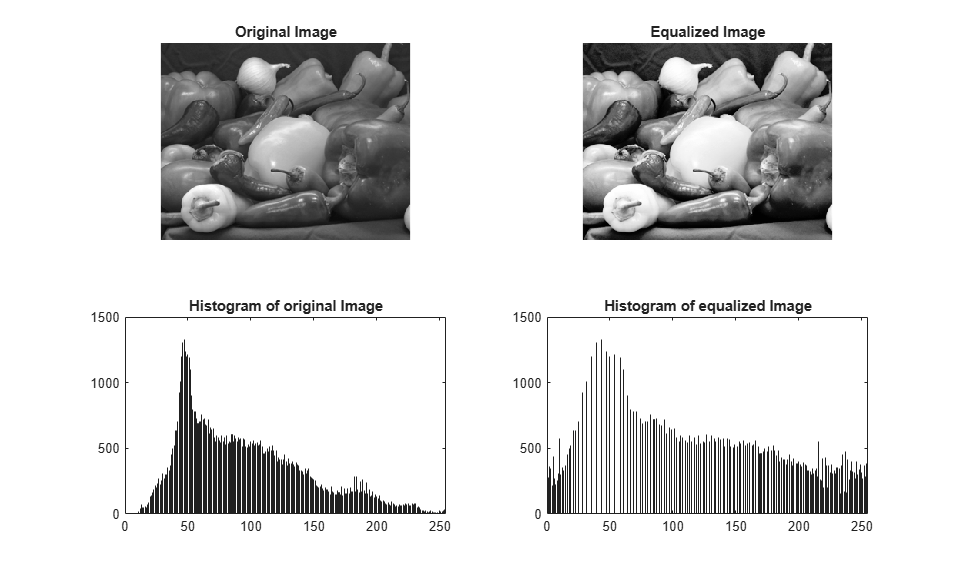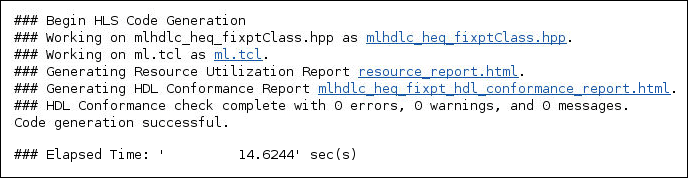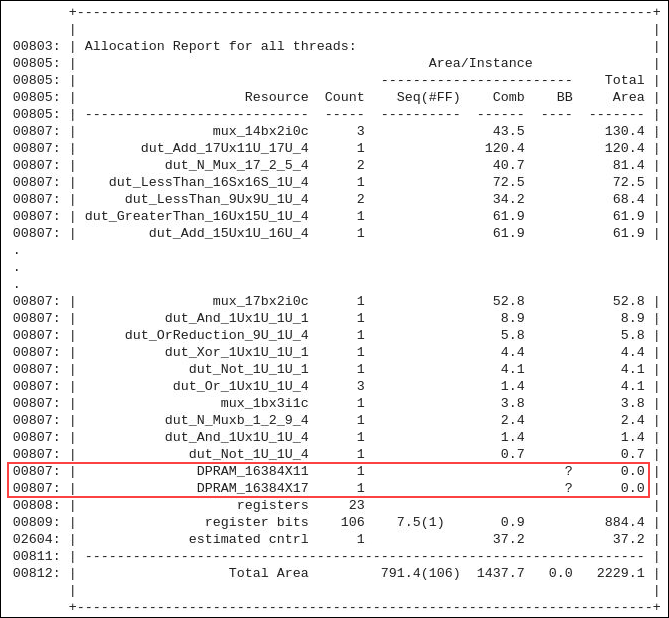# Map Persistent Variables to RAM for Histogram Equalization

This example shows how to use the RAM mapping optimization in HDL Coder™ to map persistent matrix variables to block RAMs in hardware.

### Introduction

In MATLAB, you can easily create, access, modify, and manipulate matrices.

When processing such MATLAB code, HDL Coder maps these matrices to wires or registers in hardware. For example, local temporary matrix variables are mapped to wires, whereas persistent matrix variables are mapped to registers.

The latter tends to be an inefficient mapping when the matrix size is large because the number of register resources available is limited. It also complicates synthesis, placement, and routing.

Modern FPGAs feature block RAMs that are designed to have large matrices. HDL Coder takes advantage of this feature and maps matrices to block RAMs to improve area efficiency. For certain designs, mapping these persistent matrices to RAMs is mandatory if the design is to be realized. State-of-the-art synthesis tools might not be able to synthesize designs when large matrices are mapped to registers, whereas the size is more manageable when the same matrices are mapped to RAMs.

### Algorithm

The Histogram Equalization algorithm enhances the contrast of images by transforming the values in an intensity image so that the histogram of the output image is approximately flat.

```I = imread('pout.tif'); J = histeq(I); subplot(2,2,1); imshow( I ); subplot(2,2,2); imhist(I) subplot(2,2,3); imshow( J ); subplot(2,2,4); imhist(J) ```### MATLAB Design

```design_name = 'mlhdlc_heq'; testbench_name = 'mlhdlc_heq_tb'; ```

Review the MATLAB design

```type(design_name); ```
```%%%%%%%%%%%%%%%%%%%%%%%%%%%%%%%%%%%%%%%%%%%%%%%%%%%%%%%%%%%%%%%%%%%%%%%%%%% % heq.m % Histogram Equalization Algorithm %%%%%%%%%%%%%%%%%%%%%%%%%%%%%%%%%%%%%%%%%%%%%%%%%%%%%%%%%%%%%%%%%%%%%%%%%%% function [x_out, y_out, pixel_out] = ... mlhdlc_heq(x_in, y_in, pixel_in, width, height) % Copyright 2011-2015 The MathWorks, Inc. persistent histogram persistent transferFunc persistent histInd persistent cumSum if isempty(histogram) histogram = zeros(1, 2^14); transferFunc = zeros(1, 2^14); histInd = 0; cumSum = 0; end % Figure out indexes based on where we are in the frame if y_in < height && x_in < width % valid pixel data histInd = pixel_in + 1; elseif y_in == height && x_in == 0 % first column of height+1 histInd = 1; elseif y_in >= height % vertical blanking period histInd = min(histInd + 1, 2^14); elseif y_in < height % horizontal blanking - do nothing histInd = 1; end %Read histogram (must be outside conditional logic) histValRead = histogram(histInd); %Read transfer function (must be outside conditional logic) transValRead = transferFunc(histInd); %If valid part of frame add one to pixel bin and keep transfer func val if y_in < height && x_in < width histValWrite = histValRead + 1; %Add pixel to bin transValWrite = transValRead; %Write back same value cumSum = 0; elseif y_in >= height %In blanking time index through all bins and reset to zero histValWrite = 0; transValWrite = cumSum + histValRead; cumSum = transValWrite; else histValWrite = histValRead; transValWrite = transValRead; end %Write histogram (must be outside conditional logic) histogram(histInd) = histValWrite; %Write transfer function (must be outside conditional logic) transferFunc(histInd) = transValWrite; pixel_out = transValRead; x_out = x_in; y_out = y_in; ```
```type(testbench_name); ```
```%Test bench for Histogram Equalization % Copyright 2011-2018 The MathWorks, Inc. testFile = 'mlhdlc_img_peppers.png'; imgOrig = imread(testFile); [height, width] = size(imgOrig); imgOut = zeros(height,width); hBlank = 20; % make sure we have enough vertical blanking to filter the histogram vBlank = ceil(2^14/(width+hBlank)); for frame = 1:2 disp(['working on frame: ', num2str(frame)]); for y_in = 0:height+vBlank-1 %disp(['frame: ', num2str(frame), ' of 2, row: ', num2str(y_in)]); for x_in = 0:width+hBlank-1 if x_in < width && y_in < height pixel_in = double(imgOrig(y_in+1, x_in+1)); else pixel_in = 0; end [x_out, y_out, pixel_out] = ... mlhdlc_heq(x_in, y_in, pixel_in, width, height); if x_out < width && y_out < height imgOut(y_out+1,x_out+1) = pixel_out; end end end % normalize image to 255 imgOut = round(255*imgOut/max(max(imgOut))); figure(1) subplot(2,2,1); imshow(imgOrig, [0,255]); title('Original Image'); subplot(2,2,2); imshow(imgOut, [0,255]); title('Equalized Image'); subplot(2,2,3); histogram(double(imgOrig(:)),2^14-1); axis([0, 255, 0, 1500]) title('Histogram of original Image'); subplot(2,2,4); histogram(double(imgOut(:)),2^14-1); axis([0, 255, 0, 1500]) title('Histogram of equalized Image'); end ```

### Simulate the Design

Before code generation, simulate the design by using the HDL test bench to make sure that there are no run-time errors.

```mlhdlc_heq_tb ```
```working on frame: 1 working on frame: 2 ```### Setup for the Example

To copy the required files into a temporary folder, run this code:

```mlhdlc_demo_dir = fullfile(matlabroot, 'toolbox', 'hdlcoder', 'hdlcoderdemos', 'matlabhdlcoderdemos'); mlhdlc_temp_dir = [tempdir 'mlhdlc_heq']; % create a temporary folder and copy the MATLAB files cd(tempdir); [~, ~, ~] = rmdir(mlhdlc_temp_dir, 's'); mkdir(mlhdlc_temp_dir); cd(mlhdlc_temp_dir); % copy files to the temp dir copyfile(fullfile(mlhdlc_demo_dir, [design_name,'.m*']), mlhdlc_temp_dir); copyfile(fullfile(mlhdlc_demo_dir, [testbench_name,'.m*']), mlhdlc_temp_dir); copyfile(fullfile(mlhdlc_demo_dir, 'mlhdlc_img_peppers.png'), mlhdlc_temp_dir); ```

### Create HDL Coder™ Project

```coder -hdlcoder -new mlhdlc_heq_prj ```

Add the file `mlhdlc_heq.m` to the project as the MATLAB Function and `mlhdlc_heq_tb.m` as the MATLAB Test Bench.

### SystemC Code Generation by Using RAM Mapping Optimization

To generate SystemC code from a MATLAB design:

1. At the MATLAB command line, setup the high-level synthesis (HLS) tool path for SystemC code generation by using the function `hdlsetuphlstoolpath`.

2. Start the HDL Workflow Advisor.

3. Select as MATLAB to SystemC as the Code Generation Workflow.

4. In Select Code Generation Target step, select Workflow as High Level Synthesis and Synthesis tool as Cadence Stratus.

5. To map persistent variables to block RAMs in the generated code. Select Map persistent array variables to RAMs check box in the Optimizations tab of the SystemC Code Generation step.6. Right-click SystemC Code Generation and choose the option Run to selected task to run all the steps from the beginning through the SystemC code generation.

Examine the generated SystemC code by clicking the hyperlinks in the Code Generation Log window.

### Examine the Generated Code

Examine the messages in the log window to see the RAM files generated with the design.### Examine the Resource Report

Examine the generated resource report, which shows the number of RAMs inferred, by following the `mlhdlc_heq_fixpt_syn_results.txt` link in the generated code window.

The Allocation Report highlights the variables mapped to RAM.### Additional Notes on RAM Mapping

• MATLAB functions can have any number of RAM matrices.

• All matrix variables in MATLAB that are declared persistent and meet the threshold criteria get mapped to RAMs.